# To estimate: The value of f ′ ( 0 ) using the graph of f.### Single Variable Calculus: Concepts...

4th Edition
James Stewart
Publisher: Cengage Learning
ISBN: 9781337687805### Single Variable Calculus: Concepts...

4th Edition
James Stewart
Publisher: Cengage Learning
ISBN: 9781337687805

#### Solutions

Chapter 2.7, Problem 2E

(a)

To determine

## To estimate: The value of f′(0) using the graph of f.

Expert Solution

The value of f(0) is 6.7.

### Explanation of Solution

Estimation:

Draw the tangent at x=0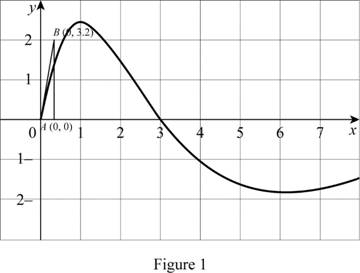The calculation of the slope at x=0 is as follows,

From Figure 1,

m=200.30=20.3=6.67

Thus, f(0)=6.67

(b)

To determine

### To estimate: The value of f′(1) using the graph of f.

Expert Solution

The value of f(1) is 0.

### Explanation of Solution

Estimation:

Draw the tangent at x=1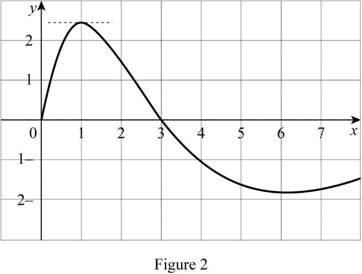From the Figure 2, it is clear that the tangent to the graph at x=1 is horizontal. So, f(1)0.

Thus, f(1)=0.

(c)

To determine

### To estimate: The value of f′(2) using the graph of f.

Expert Solution

The value of f(2) is 1.5

### Explanation of Solution

Estimation:

Draw the tangent at x=2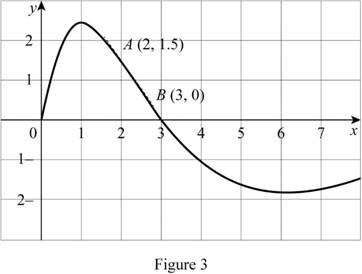Calculation:

The calculation of the slope of f at x=2 as follows,

From Figure 3,

m=01.532=1.51=1.5

So, f(2)1.5

Thus, f(2)=1.5.

(d)

To determine

### To estimate: The value of f′(3) using the graph of f.

Expert Solution

The value of f(3) is 1

### Explanation of Solution

Estimation:

Draw the tangent at x=3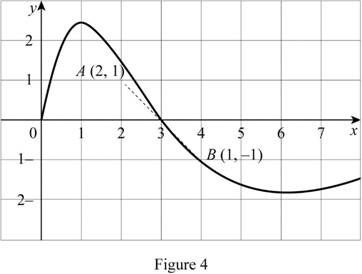From Figure 4, the estimation of the slope of f at x=3 as follows,

m=1142=22=1

So, f(3)1

Thus, f(3)=1.

(e)

To determine

### To estimate: The value of f′(4) using the graph of f.

Expert Solution

The value of f(4) is 1.

### Explanation of Solution

Estimation:

Draw the tangent at x=4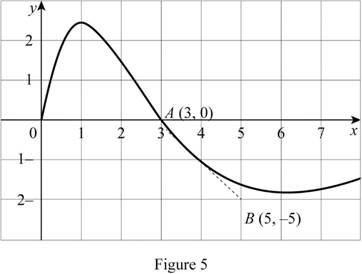From Figure 5, the calculation of the slope of f at x=4 as follows,

m=2053=22=1

So, f(4)1

Thus, f(4)=1.

(f)

To determine

### To estimate: The value of f′(5) using the graph of f.

Expert Solution

The value of f(5) is 0.2

### Explanation of Solution

Estimation:

Draw the tangent at x=5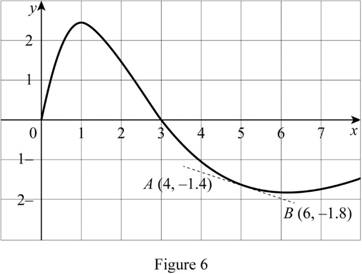From Figure 7, the calculation of the slope of f at x=5 as follows,

m=1.8+1.464=0.42=0.2

So, f(5)0.2

Thus, f(5)=0.2.

(g)

To determine

### To estimate: The value of f′(6) using the graph of f.

Expert Solution

The value of f(6) is 0.

### Explanation of Solution

Estimation:

Draw the tangent at x=6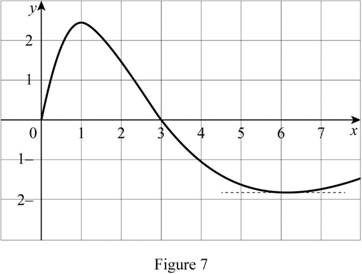From the Figure 7, it is clear that the tangent to the graph at x=6 is horizontal. So,f(6)0.

Thus, f(6)=0.

(h)

To determine

### To estimate: The value of f′(7) using the graph of f.

Expert Solution

Solution:

The value of f(7) is 0.15.

### Explanation of Solution

Estimation:

Draw the tangent at x=7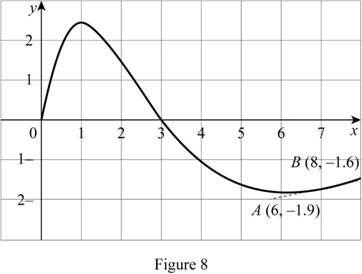The calculation of the slope of the graph at x=7 is as follows,

From the Figure 8,

m=1.6+1.986=0.32=0.15

So,f(7)0.15

Thus, f(7)=0.15

To sketch the graph of f use the information from all the above parts to draw the graph of f(x)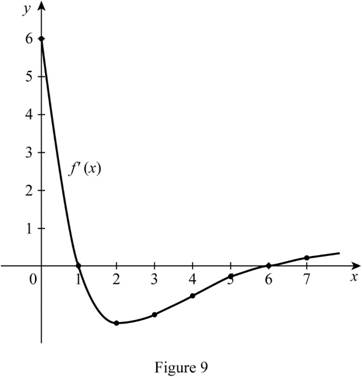From Figure 9, it is observed that the graph of f is positive on the interval (0,1)(6,7) and negative in (1,6).

### Have a homework question?

Subscribe to bartleby learn! Ask subject matter experts 30 homework questions each month. Plus, you’ll have access to millions of step-by-step textbook answers!